# GenericMap#

Bases: NDData

A Generic spatially-aware 2D data array

Parameters:
• data (numpy.ndarray, list) – A 2d list or ndarray containing the map data.

• header (dict) – A dictionary of the original image header tags.

• plot_settings (dict, optional) – Plot settings.

Other Parameters:

**kwargs – Additional keyword arguments are passed to NDData init.

Examples

>>> import sunpy.map
>>> import sunpy.data.sample
>>> aia = sunpy.map.Map(sunpy.data.sample.AIA_171_IMAGE)
>>> aia
<sunpy.map.sources.sdo.AIAMap object at ...>
SunPy Map
---------
Observatory:                 SDO
Instrument:          AIA 3
Detector:            AIA
Measurement:                 171.0 Angstrom
Wavelength:          171.0 Angstrom
Observation Date:    2011-06-07 06:33:02
Exposure Time:               0.234256 s
Dimension:           [1024. 1024.] pix
Coordinate System:   helioprojective
Scale:                       [2.402792 2.402792] arcsec / pix
Reference Pixel:     [511.5 511.5] pix
Reference Coord:     [3.22309951 1.38578135] arcsec
array([[ -95.92475  ,    7.076416 ,   -1.9656711, ..., -127.96519  ,
-127.96519  , -127.96519  ],
[ -96.97533  ,   -5.1167884,    0.       , ...,  -98.924576 ,
-104.04137  , -127.919716 ],
[ -93.99607  ,    1.0189276,   -4.0757103, ...,   -5.094638 ,
-37.95505  , -127.87541  ],
...,
[-128.01454  , -128.01454  , -128.01454  , ..., -128.01454  ,
-128.01454  , -128.01454  ],
[-127.899666 , -127.899666 , -127.899666 , ..., -127.899666 ,
-127.899666 , -127.899666 ],
[-128.03072  , -128.03072  , -128.03072  , ..., -128.03072  ,
-128.03072  , -128.03072  ]], dtype=float32)

>>> aia.spatial_units
SpatialPair(axis1=Unit("arcsec"), axis2=Unit("arcsec"))
>>> aia.peek()


Notes

A number of the properties of this class are returned as two-value named tuples that can either be indexed by position ( or ) or be accessed by the names (.x and .y) or (.axis1 and .axis2). Things that refer to pixel axes use the .x, .y convention, where x and y refer to the FITS axes (x for columns y for rows). Spatial axes use .axis1 and .axis2 which correspond to the first and second axes in the header. axis1 corresponds to the coordinate axis for x and axis2 corresponds to y.

This class assumes that the metadata adheres to the FITS 4 standard. Where the CROTA2 metadata is provided (without PC_ij) it assumes a conversion to the standard PC_ij described in section 6.1 of . Calabretta & Greisen (2002)

Warning

If a header has CD_ij values but no PC_ij values, CDELT values are required for this class to construct the WCS. If a file with more than two dimensions is feed into the class, only the first two dimensions (NAXIS1, NAXIS2) will be loaded and the rest will be discarded.

Attributes Summary

 bottom_left_coord The physical coordinate at the center of the bottom left ([0, 0]) pixel. carrington_latitude Observer Carrington latitude. carrington_longitude Observer Carrington longitude. center Return a coordinate object for the center pixel of the array. cmap Return the matplotlib.colors.Colormap instance this map uses. coordinate_frame An astropy.coordinates.BaseCoordinateFrame instance created from the coordinate information for this Map, or None if the frame cannot be determined. coordinate_system Coordinate system used for x and y axes (ctype1/2). data The stored dataset. date Image observation time. date_average Average time of the image acquisition. date_end Time of the end of the image acquisition. date_start Time of the beginning of the image acquisition. detector Detector name. dimensions The dimensions of the array (x axis first, y axis second). dsun Observer distance from the center of the Sun. dtype The numpy.dtype of the array of the map. exposure_time Exposure time of the image. fits_header A Header representation of the meta attribute. heliographic_latitude Observer heliographic latitude. heliographic_longitude Observer heliographic longitude. instrument Instrument name. latex_name LaTeX formatted description of the Map. mask Mask for the dataset, if any. measurement Measurement wavelength. meta The map metadata. name Human-readable description of the Map. ndim The value of numpy.ndarray.ndim of the data array of the map. nickname An abbreviated human-readable description of the map-type; part of the Helioviewer data model. observatory Observatory or Telescope name. observer_coordinate the property method being decorated processing_level Returns the FITS processing level if present. psf Image representation of the PSF for the dataset. quantity Unitful representation of the map data. reference_coordinate Reference point WCS axes in data units (i.e. crval1, crval2). reference_pixel Pixel of reference coordinate. rotation_matrix Matrix describing the transformation needed to align the reference pixel with the coordinate axes. rsun_meters Assumed radius of observed emission from the Sun center. rsun_obs Angular radius of the observation from Sun center. scale Image scale along the x and y axes in units/pixel (i.e. cdelt1, cdelt2). spatial_units Image coordinate units along the x and y axes (i.e. cunit1, cunit2). timeunit The Unit of the exposure time of this observation. top_right_coord The physical coordinate at the center of the the top right ([-1, -1]) pixel. uncertainty Uncertainty in the dataset, if any. unit Unit of the map data. wavelength Wavelength of the observation. waveunit The Unit of the wavelength of this observation. wcs the property method being decorated

Methods Summary

 contour(level, **kwargs) Returns coordinates of the contours for a given level value. draw_contours(levels[, axes]) Draw contours of the data. draw_grid([axes, grid_spacing, annotate, system]) Draws a coordinate overlay on the plot in the Heliographic Stonyhurst coordinate system. draw_limb([axes, resolution]) Draws the solar limb as seen by the map's observer. draw_quadrangle(bottom_left, *[, width, ...]) Draw a quadrangle defined in world coordinates on the plot using Astropy's Quadrangle. max(*args, **kwargs) Calculate the maximum value of the data array, ignoring NaNs. mean(*args, **kwargs) Calculate the mean of the data array, ignoring NaNs. min(*args, **kwargs) Calculate the minimum value of the data array, ignoring NaNs. peek([draw_limb, draw_grid, colorbar]) Displays a graphical overview of the data in this object for user evaluation. pixel_to_world(x, y) Convert a pixel coordinate to a data (world) coordinate. plot([annotate, axes, title, autoalign, ...]) Plots the map object using matplotlib, in a method equivalent to imshow() using nearest neighbor interpolation. Display a quicklook summary of the Map instance using the default web browser. reproject_to(target_wcs, *[, algorithm, ...]) Reproject the map to a different world coordinate system (WCS) resample(dimensions[, method]) Resample to new dimension sizes. rotate([angle, rmatrix, order, scale, ...]) Returns a new rotated and rescaled map. save(filepath[, filetype]) Save a map to a file. shift_reference_coord(axis1, axis2) Returns a map shifted by a specified amount to, for example, correct for a bad map location. std(*args, **kwargs) Calculate the standard deviation of the data array, ignoring NaNs. submap(bottom_left, *[, top_right, width, ...]) Returns a submap defined by a rectangle. superpixel(dimensions[, offset, func]) Returns a new map consisting of superpixels formed by applying 'func' to the original map data. world_to_pixel(coordinate) Convert a world (data) coordinate to a pixel coordinate.

Attributes Documentation

bottom_left_coord#

The physical coordinate at the center of the bottom left ([0, 0]) pixel.

carrington_latitude#

Observer Carrington latitude.

carrington_longitude#

Observer Carrington longitude.

center#

Return a coordinate object for the center pixel of the array.

If the array has an even number of pixels in a given dimension, the coordinate returned lies on the edge between the two central pixels.

cmap#

Return the matplotlib.colors.Colormap instance this map uses.

coordinate_frame#

An astropy.coordinates.BaseCoordinateFrame instance created from the coordinate information for this Map, or None if the frame cannot be determined.

coordinate_system#

Coordinate system used for x and y axes (ctype1/2).

If not present, defaults to (HPLN-TAN, HPLT-TAN), and emits a warning.

data#

The stored dataset.

Type:

ndarray-like

date#

Image observation time.

For different combinations of map metadata this can return either the start time, end time, or a time between these. It is recommended to use date_average, date_start, or date_end instead if you need one of these specific times.

Taken from, in order of preference:

1. The DATE-OBS FITS keyword

2. date_average

3. date_start

4. date_end

5. The current time

date_average#

Average time of the image acquisition.

Taken from the DATE-AVG FITS keyword if present, otherwise halfway between date_start and date_end if both pieces of metadata are present.

date_end#

Time of the end of the image acquisition.

Taken from the DATE-END FITS keyword.

date_start#

Time of the beginning of the image acquisition.

Taken from the DATE-BEG FITS keyword.

detector#

Detector name.

This is taken from the ‘DETECTOR’ FITS keyword.

dimensions#

The dimensions of the array (x axis first, y axis second).

dsun#

Observer distance from the center of the Sun.

dtype#

The numpy.dtype of the array of the map.

exposure_time#

Exposure time of the image.

This is taken from the ‘XPOSURE’ keyword or the ‘EXPTIME’ FITS keyword, in that order.

A Header representation of the meta attribute.

heliographic_latitude#

Observer heliographic latitude.

heliographic_longitude#

Observer heliographic longitude.

instrument#

Instrument name.

latex_name#

LaTeX formatted description of the Map.

Mask for the dataset, if any.

Masks should follow the numpy convention that valid data points are marked by False and invalid ones with True.

Type:

any type

measurement#

Measurement wavelength.

This is taken from the ‘WAVELNTH’ FITS keywords. If the keyword is not present, defaults to None. If ‘WAVEUNIT’ keyword isn’t present, defaults to dimensionless units.

meta#

This is used to interpret the map data. It may have been modified from the original metadata by sunpy. See the added_items, removed_items and modified_items properties of MetaDict to query how the metadata has been modified.

name#

ndim#

The value of numpy.ndarray.ndim of the data array of the map.

nickname#

An abbreviated human-readable description of the map-type; part of the Helioviewer data model.

observatory#

Observatory or Telescope name.

This is taken from the ‘OBSRVTRY’ FITS keyword.

observer_coordinate#

the property method being decorated

Type:

prop

processing_level#

Returns the FITS processing level if present.

This is taken from the ‘LVL_NUM’ FITS keyword.

psf#
quantity#

Unitful representation of the map data.

reference_coordinate#

Reference point WCS axes in data units (i.e. crval1, crval2). This value includes a shift if one is set.

reference_pixel#

Pixel of reference coordinate.

The pixel returned uses zero-based indexing, so will be 1 pixel less than the FITS CRPIX values.

rotation_matrix#

Matrix describing the transformation needed to align the reference pixel with the coordinate axes.

The order or precedence of FITS keywords which this is taken from is: - PC*_* - CD*_* - CROTA*

Notes

In many cases this is a simple rotation matrix, hence the property name. It general it does not have to be a pure rotation matrix, and can encode other transformations e.g., skews for non-orthgonal coordinate systems.

rsun_meters#

Assumed radius of observed emission from the Sun center.

This is taken from the RSUN_REF FITS keyword, if present. If not, and angular radius metadata is present, it is calculated from rsun_obs and dsun. If neither pieces of metadata are present, defaults to the standard photospheric radius.

rsun_obs#

Angular radius of the observation from Sun center.

This value is taken (in order of preference) from the ‘RSUN_OBS’, ‘SOLAR_R’, or ‘RADIUS’ FITS keywords. If none of these keys are present, the angular radius is calculated from rsun_meters and dsun.

scale#

Image scale along the x and y axes in units/pixel (i.e. cdelt1, cdelt2).

If the CDij matrix is defined but no CDELTi values are explicitly defined, effective CDELTi values are constructed from the CDij matrix. The effective CDELTi values are chosen so that each row of the PCij matrix has unity norm. This choice is optimal if the PCij matrix is a pure rotation matrix, but may not be as optimal if the PCij matrix includes any skew.

spatial_units#

Image coordinate units along the x and y axes (i.e. cunit1, cunit2).

timeunit#

The Unit of the exposure time of this observation.

Taken from the “TIMEUNIT” FITS keyword, and defaults to seconds (as per) the FITS standard).

top_right_coord#

The physical coordinate at the center of the the top right ([-1, -1]) pixel.

uncertainty#

Uncertainty in the dataset, if any.

Should have an attribute uncertainty_type that defines what kind of uncertainty is stored, such as 'std' for standard deviation or 'var' for variance. A metaclass defining such an interface is NDUncertainty but isn’t mandatory.

Type:

any type

unit#

Unit of the map data.

This is taken from the ‘BUNIT’ FITS keyword. If no ‘BUNIT’ entry is present in the metadata then this returns None. If the ‘BUNIT’ value cannot be parsed into a unit a warning is raised, and None returned.

wavelength#

Wavelength of the observation.

This is taken from the ‘WAVELNTH’ FITS keywords. If the keyword is not present, defaults to None. If ‘WAVEUNIT’ keyword isn’t present, defaults to dimensionless units.

waveunit#

The Unit of the wavelength of this observation.

This is taken from the ‘WAVEUNIT’ FITS keyword. If the keyword is not present, defaults to None

wcs#

the property method being decorated

Type:

prop

Methods Documentation

contour(level, **kwargs)[source]#

Returns coordinates of the contours for a given level value.

For details of the contouring algorithm see skimage.measure.find_contours.

Parameters:
Returns:

contours (list of (n,2) SkyCoord) – Coordinates of each contour.

Examples

>>> import astropy.units as u
>>> import sunpy.map
>>> import sunpy.data.sample
>>> aia = sunpy.map.Map(sunpy.data.sample.AIA_171_IMAGE)
>>> contours = aia.contour(50000 * u.ct)
>>> print(contours)
<SkyCoord (Helioprojective: obstime=2011-06-07T06:33:02.770, rsun=696000.0 km, observer=<HeliographicStonyhurst Coordinate (obstime=2011-06-07T06:33:02.770, rsun=696000.0 km): (lon, lat, radius) in (deg, deg, m)
(-0.00406308, 0.04787238, 1.51846026e+11)>): (Tx, Ty) in arcsec
[(719.59798458, -352.60839064), (717.19243987, -353.75348121),
...

draw_contours(levels, axes=None, **contour_args)[source]#

Draw contours of the data.

Parameters:
Returns:

cs (list) – The QuadContourSet object, after it has been added to axes.

Notes

Extra keyword arguments to this function are passed through to the contour function.

draw_grid(axes=None, grid_spacing: Unit("deg") = <Quantity 15. deg>, annotate=True, system='stonyhurst', **kwargs)[source]#

Draws a coordinate overlay on the plot in the Heliographic Stonyhurst coordinate system.

To overlay other coordinate systems see the WCSAxes Documentation

Parameters:
Returns:

overlay (CoordinatesMap) – The wcsaxes coordinate overlay instance.

Notes

Keyword arguments are passed onto the sunpy.visualization.wcsaxes_compat.wcsaxes_heliographic_overlay function.

draw_limb(axes=None, *, resolution=1000, **kwargs)[source]#

Draws the solar limb as seen by the map’s observer.

The limb is a circle for only the simplest plots. If the coordinate frame of the limb is different from the coordinate frame of the plot axes, not only may the limb not be a true circle, a portion of the limb may be hidden from the observer. In that case, the circle is divided into visible and hidden segments, represented by solid and dotted lines, respectively.

Parameters:
• axes (axes or None) – Axes to plot limb on or None to use current axes.

• resolution (int) – The number of points to use to represent the limb.

Returns:

Notes

Keyword arguments are passed onto the patches.

If the limb is a true circle, visible will instead be Circle and hidden will be None. If there are no visible points (e.g., on a synoptic map any limb is fully) visible hidden will be None.

To avoid triggering Matplotlib auto-scaling, these patches are added as artists instead of patches. One consequence is that the plot legend is not populated automatically when the limb is specified with a text label. See Composing Custom Legends in the Matplotlib documentation for examples of creating a custom legend.

draw_quadrangle(bottom_left, *, width: (Unit('deg'), Unit('pix')) = None, height: (Unit('deg'), Unit('pix')) = None, axes=None, top_right=None, **kwargs)[source]#

Draw a quadrangle defined in world coordinates on the plot using Astropy’s Quadrangle.

This draws a quadrangle that has corners at (bottom_left, top_right), and has sides aligned with the coordinate axes of the frame of bottom_left, which may be different from the coordinate axes of the map.

If width and height are specified, they are respectively added to the longitude and latitude of the bottom_left coordinate to calculate a top_right coordinate.

Parameters:
Returns:

quad (Quadrangle) – The added patch.

Notes

Extra keyword arguments to this function are passed through to the Quadrangle instance.

Examples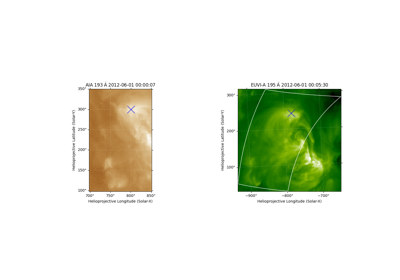AIA to STEREO coordinate conversion

AIA to STEREO coordinate conversion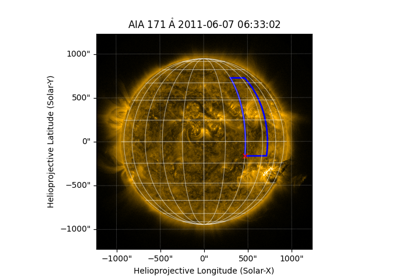Drawing a rectangle on a map

Drawing a rectangle on a map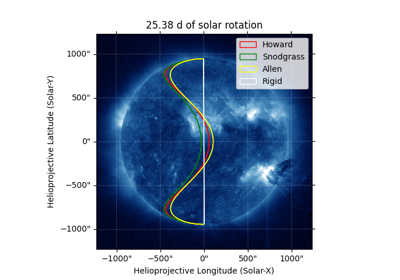Comparing differential-rotation models

Comparing differential-rotation models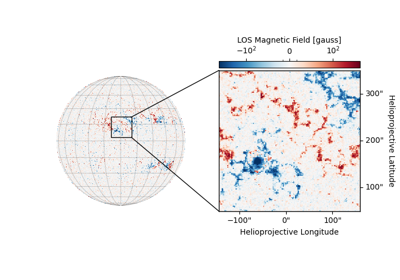HMI Showcase: Cutout

HMI Showcase: Cutout
max(*args, **kwargs)[source]#

Calculate the maximum value of the data array, ignoring NaNs.

mean(*args, **kwargs)[source]#

Calculate the mean of the data array, ignoring NaNs.

min(*args, **kwargs)[source]#

Calculate the minimum value of the data array, ignoring NaNs.

peek(draw_limb=False, draw_grid=False, colorbar=True, **matplot_args)[source]#

Displays a graphical overview of the data in this object for user evaluation. For the creation of plots, users should instead use the plot method and Matplotlib’s pyplot framework.

Parameters:
• draw_limb (bool) – Whether the solar limb should be plotted.

• draw_grid (bool or Quantity) – Whether solar meridians and parallels are plotted. If Quantity then sets degree difference between parallels and meridians.

• colorbar (bool) – Whether to display a colorbar next to the plot.

• **matplot_args (dict) – Matplotlib Any additional imshow arguments that should be used when plotting.

pixel_to_world(x: Unit('pix'), y: Unit('pix'))[source]#

Convert a pixel coordinate to a data (world) coordinate.

Parameters:
Returns:

coord (astropy.coordinates.SkyCoord) – A coordinate object representing the output coordinate.

plot(annotate=True, axes=None, title=True, autoalign=False, clip_interval: Unit('%') = None, **imshow_kwargs)[source]#

Plots the map object using matplotlib, in a method equivalent to imshow() using nearest neighbor interpolation.

Parameters:

Examples

>>> # Simple Plot with color bar
>>> aia.plot()
>>> plt.colorbar()
>>> # Add a limb line and grid
>>> aia.plot()
>>> aia.draw_limb()
>>> aia.draw_grid()


Notes

The autoalign functionality is computationally intensive. If the plot will be interactive, the alternative approach of preprocessing the map (e.g., de-rotating it) to match the desired axes will result in better performance.

When combining autoalign functionality with Helioprojective coordinates, portions of the map that are beyond the solar disk may not appear, which may also inhibit Matplotlib’s autoscaling of the plot limits. The plot limits can be set manually. To preserve the off-disk parts of the map, using the assume_spherical_screen() context manager may be appropriate.

quicklook()[source]#

Display a quicklook summary of the Map instance using the default web browser.

Notes

The image colormap uses histogram equalization.

Clicking on the image to switch between pixel space and coordinate space requires Javascript support to be enabled in the web browser.

Examples

>>> from sunpy.map import Map
>>> import sunpy.data.sample
>>> smap = Map(sunpy.data.sample.AIA_171_IMAGE)
>>> smap.quicklook()


(which will open the following content in the default web browser)

<sunpy.map.sources.sdo.AIAMap object at 0x7f2de9b91b40>
Observatory SDO AIA 3 AIA 171.0 Angstrom 171.0 Angstrom 2011-06-07 06:33:02 0.234256 s [1024. 1024.] pix helioprojective [2.402792 2.402792] arcsec / pix [511.5 511.5] pix [3.22309951 1.38578135] arcsec
Image colormap uses histogram equalization
Click on the image to toggle between units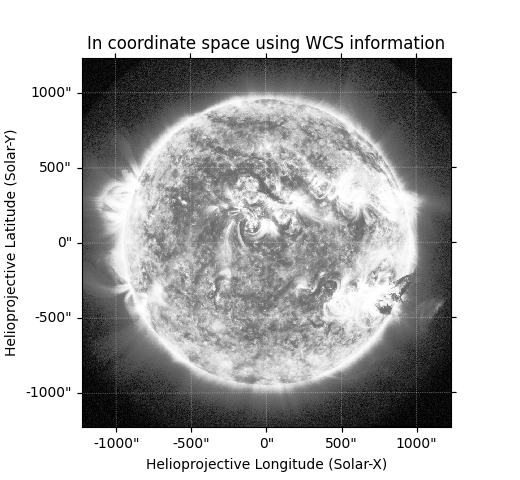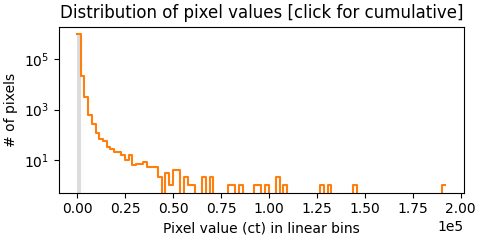reproject_to(target_wcs, *, algorithm='interpolation', return_footprint=False, **reproject_args)[source]#

Reproject the map to a different world coordinate system (WCS)

Note

This method requires the optional package reproject to be installed.

Additional keyword arguments are passed through to the reprojection function.

Parameters:
Returns:

• outmap (GenericMap) – The reprojected map

• footprint (ndarray) – Footprint of the input arary in the output array. Values of 0 indicate no coverage or valid values in the input image, while values of 1 indicate valid values. Intermediate values indicate partial coverage. Only returned if return_footprint is True.

Notes

The reprojected map does not preserve any metadata beyond the WCS-associated metadata.

The supported reproject algorithms are:

See the respective documentation for these functions for additional keyword arguments that are allowed.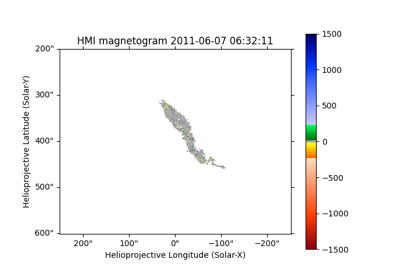Masking HMI based on the intensity of AIA

Masking HMI based on the intensity of AIA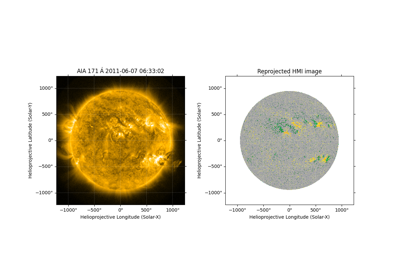Aligning AIA and HMI Data with Reproject

Aligning AIA and HMI Data with Reproject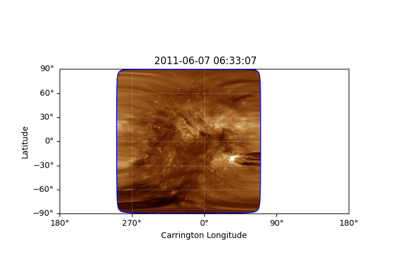Creating Carrington Maps

Creating Carrington Maps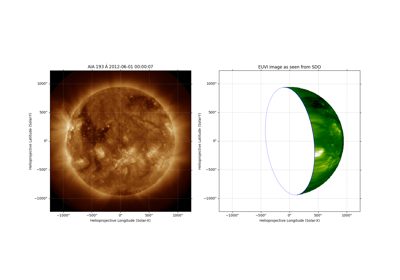Reprojecting Images to Different Observers

Reprojecting Images to Different Observers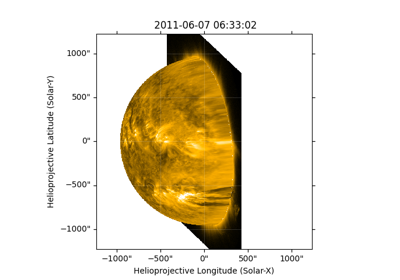Reprojecting Using a Spherical Screen

Reprojecting Using a Spherical Screen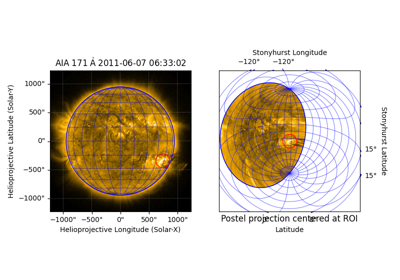Reprojecting to a Map Projection with a Custom Origin

Reprojecting to a Map Projection with a Custom OriginBlending maps using mplcairo

Blending maps using mplcairo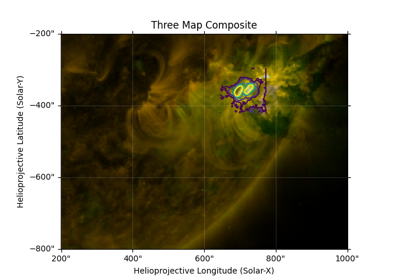Creating a Composite Plot with Three Maps

Creating a Composite Plot with Three Maps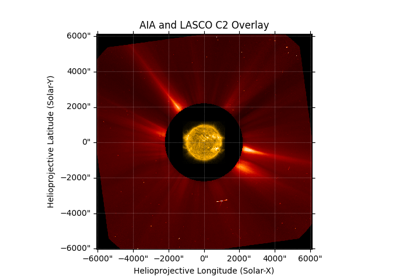Overlay an AIA image on a LASCO C2 coronagraph

Overlay an AIA image on a LASCO C2 coronagraph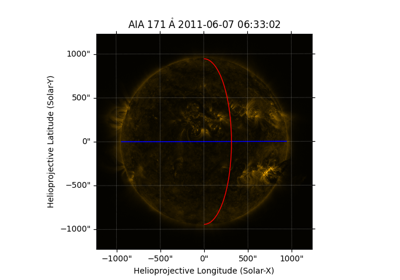Plotting the solar equator and prime meridian

Plotting the solar equator and prime meridian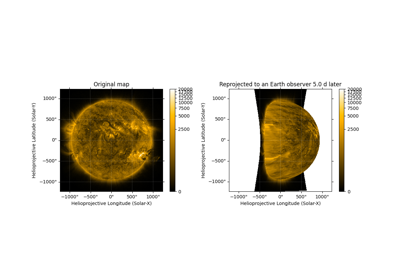Differentially rotating a map

Differentially rotating a map
resample(dimensions: Unit('pix'), method='linear')[source]#

Resample to new dimension sizes.

Uses the same parameters and creates the same coordinate lookup points as IDL’’s congrid routine, which apparently originally came from a VAX/VMS routine of the same name.

Parameters:
• dimensions (Quantity) – Output pixel dimensions. The first argument corresponds to the ‘x’ axis and the second argument corresponds to the ‘y’ axis.

• method (str) –

Method to use for resampling interpolation.
Returns:

out (GenericMap or subclass) – Resampled map

References

Rebinning

rotate(angle: Unit('deg') = None, rmatrix=None, order=3, scale=1.0, recenter=False, missing=nan, *, method='scipy', clip=True)[source]#

Returns a new rotated and rescaled map.

Specify either a rotation angle or a rotation matrix, but not both. If neither an angle or a rotation matrix are specified, the map will be rotated by the rotation information in the metadata, which should derotate the map so that the pixel axes are aligned with world-coordinate axes.

Parameters:
• angle (Quantity) – The angle (degrees) to rotate counterclockwise.

• rmatrix (array-like) – 2x2 linear transformation rotation matrix.

• order (int) – Interpolation order to be used. The precise meaning depends on the rotation method specified by method. Default: 3

• scale (float) – A scale factor for the image, default is no scaling

• recenter (bool) – If True, position the reference coordinate at the center of the new map Default: False

• missing (float) – The value to use for pixels in the output map that are beyond the extent of the input map. Default: numpy.nan

• method ({'scipy', 'scikit-image', 'opencv'}, optional) – Rotation function to use. Defaults to 'scipy'.

• clip (bool, optional) – If True, clips the pixel values of the output image to the range of the input image (including the value of missing, if used). Defaults to True.

Returns:

out (GenericMap or subclass) – A new Map instance containing the rotated and rescaled data of the original map.

sunpy.image.transform.affine_transform

The routine this method calls for the rotation.

Notes

The rotation information in the metadata of the new map is modified appropriately from that of the original map to account for the applied rotation. It will solely be in the form of a PCi_j matrix, even if the original map used the CROTA2 keyword or a CDi_j matrix.

If the map does not already contain pixels with numpy.nan values, setting missing to an appropriate number for the data (e.g., zero) will reduce the computation time.

For each NaN pixel in the input image, one or more pixels in the output image will be set to NaN, with the size of the pixel region affected depending on the interpolation order. All currently implemented rotation methods require a convolution step to handle image NaNs. This convolution normally uses scipy.signal.convolve2d(), but if OpenCV is installed, the faster cv2.filter2D() is used instead.

See sunpy.image.transform.affine_transform() for details on each of the rotation functions.

save(filepath, filetype='auto', **kwargs)[source]#

Save a map to a file.

Parameters:
• filepath (str) – Location to save file to.

• filetype (str, optional) – Any supported file extension, defaults to "auto".

• hdu_type (ExtensionHDU instance or class, optional) – By default, a FITS file is written with the map in its primary HDU. If a type is given, a new HDU of this type will be created. If a HDU instance is given, its data and header will be updated from the map. Then that HDU instance will be written to the file. The example below uses astropy.io.fits.CompImageHDU to compress the map.

• kwargs – Any additional keyword arguments are passed to write_file.

Notes

Saving with the jp2 extension will write a modified version of the given data casted to uint8 values in order to support the JPEG2000 format.

Examples

>>> from astropy.io.fits import CompImageHDU
>>> from sunpy.map import Map
>>> import sunpy.data.sample
>>> aia_map = Map(sunpy.data.sample.AIA_171_IMAGE)
>>> aia_map.save("aia171.fits", hdu_type=CompImageHDU)

shift_reference_coord(axis1: Unit('deg'), axis2: Unit('deg'))[source]#

Returns a map shifted by a specified amount to, for example, correct for a bad map location. These values are applied directly to the reference_coordinate. To check how much the reference coordinate has been modified, see sunpy.map.GenericMap.meta.modified_items['CRVAL1'] and sunpy.map.GenericMap.meta.modified_items['CRVAL2'].

Parameters:
Returns:

out (GenericMap or subclass) – A new shifted Map.

std(*args, **kwargs)[source]#

Calculate the standard deviation of the data array, ignoring NaNs.

submap(bottom_left, *, top_right=None, width: (Unit('deg'), Unit('pix')) = None, height: (Unit('deg'), Unit('pix')) = None)[source]#

Returns a submap defined by a rectangle.

Any pixels which have at least part of their area inside the rectangle are returned. If the rectangle is defined in world coordinates, the smallest array which contains all four corners of the rectangle as defined in world coordinates is returned.

Parameters:
Returns:

out (GenericMap or subclass) – A new map instance is returned representing to specified sub-region.

Notes

When specifying pixel coordinates, they are specified in Cartesian order not in numpy order. So, for example, the bottom_left= argument should be [left, bottom].

Examples

>>> import astropy.units as u
>>> from astropy.coordinates import SkyCoord
>>> import sunpy.map
>>> import sunpy.data.sample
>>> aia = sunpy.map.Map(sunpy.data.sample.AIA_171_IMAGE)
>>> bl = SkyCoord(-300*u.arcsec, -300*u.arcsec, frame=aia.coordinate_frame)
>>> tr = SkyCoord(500*u.arcsec, 500*u.arcsec, frame=aia.coordinate_frame)
>>> aia.submap(bl, top_right=tr)
<sunpy.map.sources.sdo.AIAMap object at ...>
SunPy Map
---------
Observatory:         SDO
Instrument:          AIA 3
Detector:            AIA
Measurement:         171.0 Angstrom
Wavelength:          171.0 Angstrom
Observation Date:    2011-06-07 06:33:02
Exposure Time:       0.234256 s
Dimension:           [335. 335.] pix
Coordinate System:   helioprojective
Scale:               [2.402792 2.402792] arcsec / pix
Reference Pixel:     [126.5 125.5] pix
Reference Coord:     [3.22309951 1.38578135] arcsec
...

>>> aia.submap([0,0]*u.pixel, top_right=[5,5]*u.pixel)
<sunpy.map.sources.sdo.AIAMap object at ...>
SunPy Map
---------
Observatory:         SDO
Instrument:          AIA 3
Detector:            AIA
Measurement:         171.0 Angstrom
Wavelength:          171.0 Angstrom
Observation Date:    2011-06-07 06:33:02
Exposure Time:       0.234256 s
Dimension:           [6. 6.] pix
Coordinate System:   helioprojective
Scale:               [2.402792 2.402792] arcsec / pix
Reference Pixel:     [511.5 511.5] pix
Reference Coord:     [3.22309951 1.38578135] arcsec
...

>>> width = 10 * u.arcsec
>>> height = 10 * u.arcsec
>>> aia.submap(bl, width=width, height=height)
<sunpy.map.sources.sdo.AIAMap object at ...>
SunPy Map
---------
Observatory:         SDO
Instrument:          AIA 3
Detector:            AIA
Measurement:         171.0 Angstrom
Wavelength:          171.0 Angstrom
Observation Date:    2011-06-07 06:33:02
Exposure Time:       0.234256 s
Dimension:           [5. 5.] pix
Coordinate System:   helioprojective
Scale:               [2.402792 2.402792] arcsec / pix
Reference Pixel:     [125.5 125.5] pix
Reference Coord:     [3.22309951 1.38578135] arcsec
...

>>> bottom_left_vector = SkyCoord([0, 10]  * u.deg, [0, 10] * u.deg, frame='heliographic_stonyhurst')
>>> aia.submap(bottom_left_vector)
<sunpy.map.sources.sdo.AIAMap object at ...>
SunPy Map
---------
Observatory:         SDO
Instrument:          AIA 3
Detector:            AIA
Measurement:         171.0 Angstrom
Wavelength:          171.0 Angstrom
Observation Date:    2011-06-07 06:33:02
Exposure Time:       0.234256 s
Dimension:           [70. 69.] pix
Coordinate System:   helioprojective
Scale:               [2.402792 2.402792] arcsec / pix
Reference Pixel:     [1.5 0.5] pix
Reference Coord:     [3.22309951 1.38578135] arcsec
...

superpixel(dimensions: Unit("pix"), offset: Unit("pix") = <Quantity [0., 0.] pix>, func=<function sum>)[source]#

Returns a new map consisting of superpixels formed by applying ‘func’ to the original map data.

Parameters:
• dimensions (tuple) – One superpixel in the new map is equal to (dimension, dimension) pixels of the original map. The first argument corresponds to the ‘x’ axis and the second argument corresponds to the ‘y’ axis. If non-integer values are provided, they are rounded using int.

• offset (tuple) – Offset from (0,0) in original map pixels used to calculate where the data used to make the resulting superpixel map starts. If non-integer value are provided, they are rounded using int.

• func – Function applied to the original data. The function ‘func’ must take a numpy array as its first argument, and support the axis keyword with the meaning of a numpy axis keyword (see the description of sum for an example.) The default value of ‘func’ is sum; using this causes superpixel to sum over (dimension, dimension) pixels of the original map.

Returns:

out (GenericMap or subclass) – A new Map which has superpixels of the required size.

References

world_to_pixel(coordinate)[source]#

Convert a world (data) coordinate to a pixel coordinate.

Parameters:

coordinate (SkyCoord or BaseCoordinateFrame) – The coordinate object to convert to pixel coordinates.

Returns: